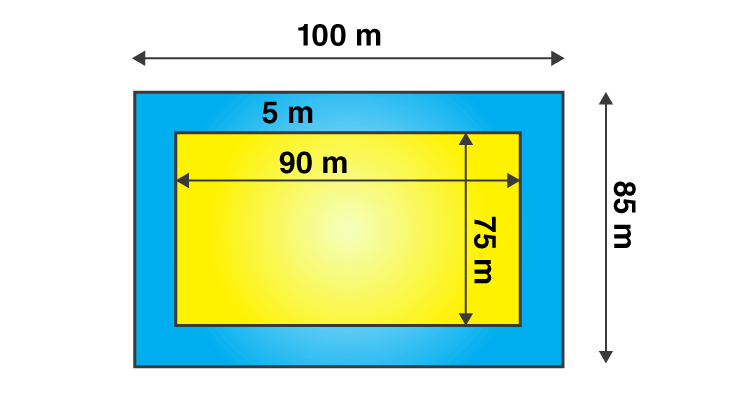# A garden is 90 m long and 75 m broad. A path 5 m wide is to be built outside and around it. Find the area of the path. Also find the area of the garden in hectare.Given

From the given data we get to know that

Length of the garden (L) = 90 m

Breadth of the garden (B) = 75 m

Find out

We have to determine the area of the garden in hectare and also the area of the path.

Solution

The garden is in the shape of a rectangle

We know that area of the rectangle is given by

= 90 × 75

= 6750 m2

From the figure, we know that

The new length and breadth of the garden when the path is included is 100 m and 85 m respectively.

A new area of the garden = 100 × 85

= 8500 m2

The area of path = New area of the garden including path – Area of garden

= 8500 – 6750

= 1750 m2

For 1 hectare = 10000 m2

Hence, area of the garden in hectare = 6750/10000

= 0.675 hectare

Area of garden in hectare=0.675 hectare

Are of the path=1750 m2(0)(0)